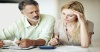Questions? 1.800.239.0356

X
1.800.239.0356# Fixed Indexed Annuities

## Fixed Indexed Annuities

• Primary Benefit:
• Secondary Benefit:
• Guarantee Future Income

Fixed indexed annuities, formerly called equity indexed annuities, are a type of deferred annuity that credits interest based on the changes to a market index, such as the S&P 500 or Dow Jones Industrial Average. Interest is credited when the index value increases, but the interest rate is guaranteed never to be less than zero, even if the market goes down. Your principal, as well as all previously credited interest earnings, can never be lost and are always protected from any unforeseen downturn in the market.

Watch this short video which provides a good overview of indexed annuities and describes how they can help you avoid market volatility, having a positive impact on your retirement savings:Insurance companies use a variety of formulas, depending on the design of a particular annuity, to determine how a change in the index correlates to the amount of interest that will be credited at the end of each index term (most commonly on an annual basis). The formula used usually consists of two parts, the crediting method and a limiting factor.

##### Frequently Used Crediting Methods

Annual Point-to-Point – Measures the percentage change in the underlying index value between two dates, at the beginning and the end of the annuity contract year.

Multi-Year Point-to-Point – Measures the percentage change in the underlying index value between two dates that are more than one year apart.

Monthly Point-to-Point – Measures the percentage change in the underlying index value each month. Usually, each monthly change is limited by a cap for positive changes, but not for negative changes. At the end of each index term, all of the recorded monthly percentage changes (both positive and negative) are added together.

Monthly Averaging – Calculated by comparing the underlying index value on the first day of the index term to the monthly average of that same index at the end of the index term. The monthly average index value equals the sum of the monthly index values recorded each month over the course of the preceding index term, divided by the number of months in that term. At the end of each index term, the ending monthly average index value is compared with the starting index value of that term.

Daily Averaging – Calculated by comparing the underlying index value on the first day of the index term to the daily average (usually 252 trading days) of that same index at the end of the index term. The daily average index value equals the sum of the daily index values recorded each trading day over the course of the preceding index term, divided by the number of trading days in that term. At the end of each index term, the ending daily average index value is compared with the starting index value of that term.

##### Common Limiting Factors

All fixed indexed annuity interest crediting formulas have some type of limiting factor that is applied to cause interest earnings to be based on only a portion of the change in the market index over the index term. In other words, in exchange for the added guarantees and principal protection, you will not receive 100% of the index market gains.

Cap Rate – An upper limit on the index-linked interest rate that is applied to the annuity. The cap rate is the maximum rate of interest the annuity can earn during the index term.

Participation Rate – Determines what percentage of the increase in the underlying market index will be used to calculate the index-linked interest credits during the index term.

Spread Rate or Margin – A specified percentage that is deducted from the total calculated change in the underlying index value to determine the net amount of index-linked interest that is credited to the annuity.